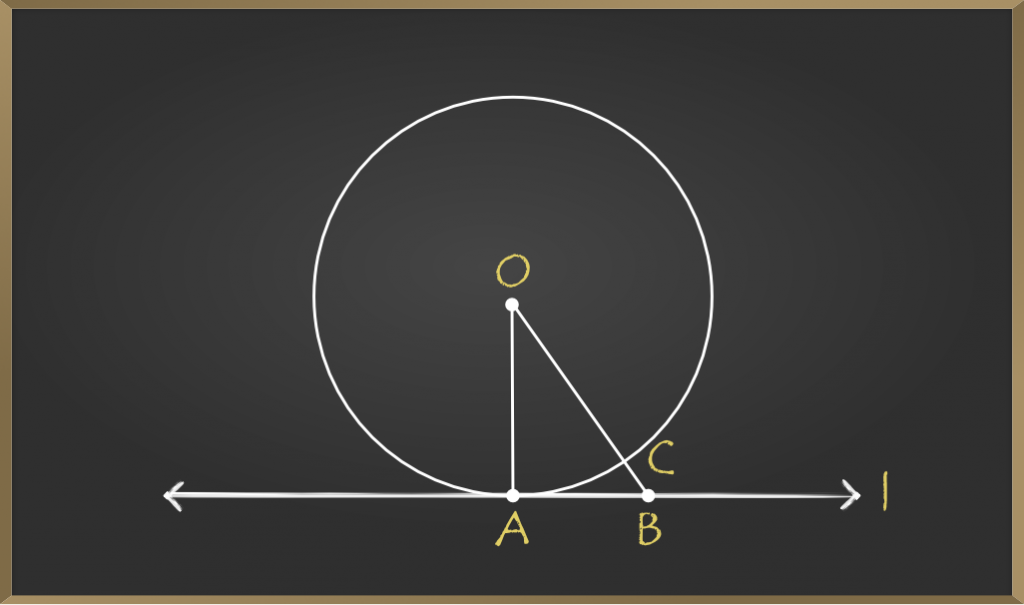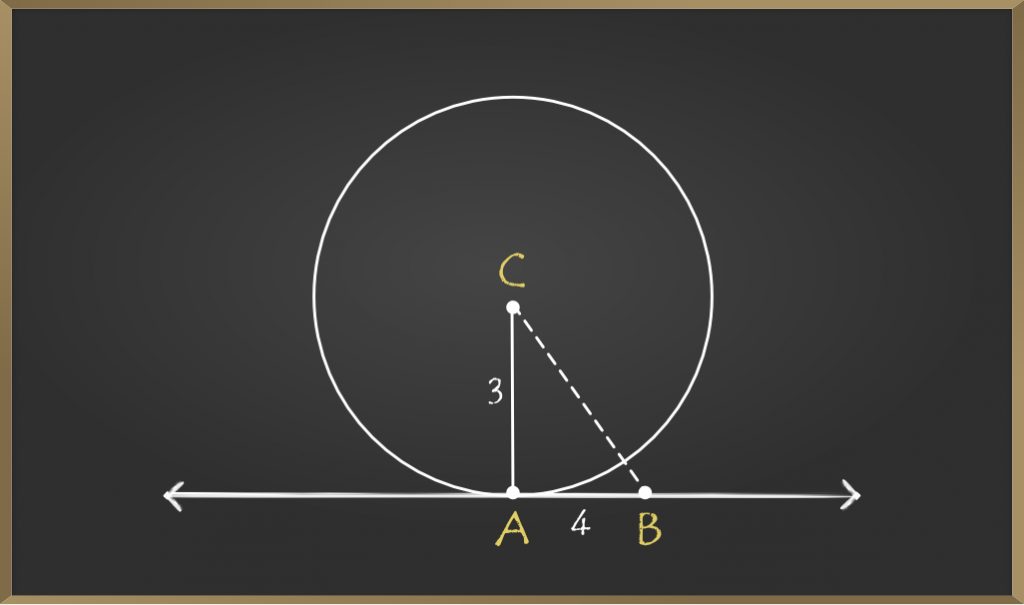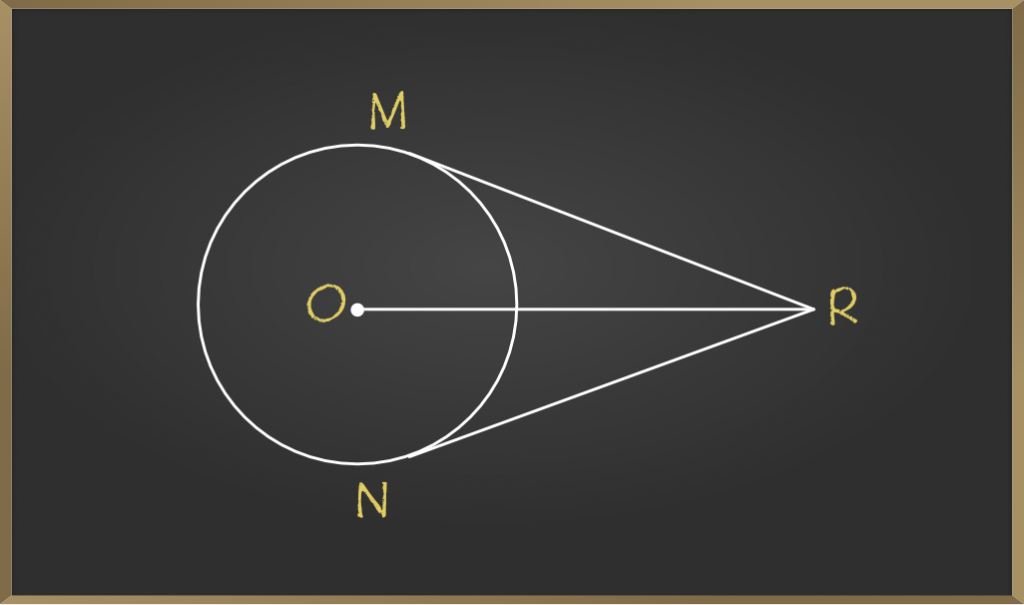GeeksforGeeks App
Open AppBrowser
Continue

# Theorem – The tangent at any point of a circle is perpendicular to the radius through the point of contact – Circles | Class 10 Maths

Tangent is a straight line drawn from an external point that touches a circle at exactly one point on the circumference of the circle. There can be an infinite number of tangents of a circle. These tangents follow certain properties that can be used as identities to perform mathematical computations on circles.

Here, in this article, we will learn about one of such properties i.e. the tangent at any point of a circle is perpendicular to the radius through the point of contact.

### To Prove: The tangent at any point of a circle is perpendicular to the radius through the point of contact

Let there be a circle C (0, r) and a tangent l at point A### Construction

Step 1: Take any point B online l, other than A.

Step 2: Join OB.

Step 3: Let us say that OB meets the circle in C.

### Proof

From prior knowledge, We know that, among all line segments joining the point O i.e. centre of the circle to a point on l (l is the tangent to the circle), the perpendicular is shortest to l.

O is the centre of the circle and the radius of the circle will be of fixed length hence we can say that:

Also OB = OC + BC.

So OC < OB.

⇒ OA < OB (since OA = OC).

The same will be the case for all other points on the tangent (l).

So OA is shorter than any other line segment joining O to any point on l.

Hence, OA ⊥ l

Hence, the tangent at any point of a circle is perpendicular to the radius through the point of contact.

### Sample Problems based on the Theorem

Problem 1: Given a circle with centre O.Two Tangent from external point P is drawn to the given circle. Find the sum of angles formed between both radius and the angles between both the tangents of the circle.

Solution:

The angles formed between the tangents and the radii is 90 degree

Since the sum of angles of the quadrilaterals is 360 degrees. And we have two 90 degrees angles formed within it.

Hence the remaining sum of angles i.e sum of angles formed between both radius and the angles between both the tangents is 360-180=180 degrees

Problem 2: Find the angle ∠CBA given that CA is a line drawn from the centre to the tangent on the circle. The length of the Radius and the base length are mentioned in the question.Solution:

It is given the line is from the centre to the tangent, so we conclude that it is at the right angle from the theorem.

Hence, we can apply trigonometric formulas to get the ∠CBA

tan(∠CBA) = CA/AB
tan(∠CBA) = 3/4

∠CBA=37 degree

Problem 3: In the figure given, O is the centre of the circle. From point R outside the circle, as shown, RM and RN are tangent touching the circle at M and N. If the length of OR = 10 cm and radius of the circle = 5 cm, then What is the length of each tangent?Solution:

Given that OM = 5 cm and OR = 10 cm
In right ∆OMR,

OR^2=OM^2+MR^2
⇒MR^2=OR^2−OM^2
⇒MR^2=100−25
MR=5√3 cm.
Also NR=5√3 cm.

Hence the length of each tangent is 5√3 cm.

My Personal Notes arrow_drop_up
Related Tutorials